john@email.johncon.com http://www.johncon.com/john/

## US GDP Historical Economic Business Cycle

The business cycle has perplexed economists, (Marx was obsessed with it,) for generations-with cyclic phenomena in the GDP estimated to be as few as 3 years, to as many as 60. But stochastic systems, such as geometric Brownian motion, have ups and downs, too, and the median duration of these ups and downs are, relatively, consistent with the estimates from the "prevailing wisdom," (to quote Keynes,) of the economists-and offers a rationale for the wide spread of economic estimates.

## US Business Cycle

The analysis of the US GDP will include various eras between 1792 and the present.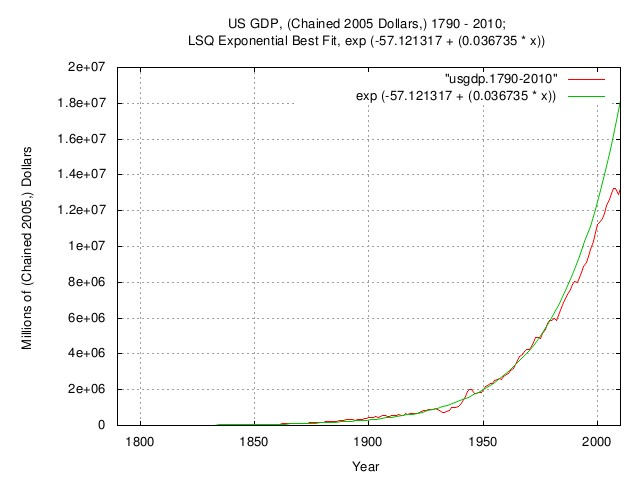Figure I

Figure I is a plot of the US GDP, chained 2005 dollars, 1790 - 2010; and the 1790 - 2010, LSQ median. (Note that median, in this case, means half of the time the empirical data is above the LSQ median, and half of the time, below.) Historically, the US GDP's annual rate of increase averaged about 3.7%. About 2% was due to the rate of increase of the population, and, about 1.7%. due to the rate of increase in productivity.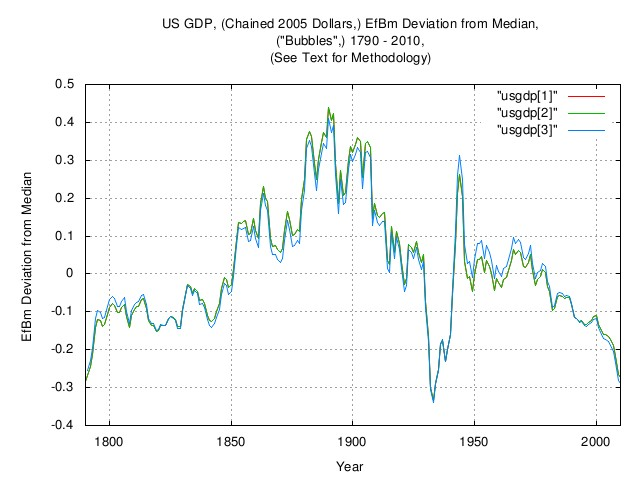Figure II

Figure II is a plot of the EfBm deviation, ("Bubbles,") of the US GDP, chained 2005 dollars, 1790 - 2010. If the US GDP was a perfect exponential, the graph would be a straight line at zero. The graph is the deviation from the straight line at zero, i.e., the productivity "Bubbles".

The huge productivity "Bubble" of the Gilded Age, (about 1850 to 1930,) is clearly visible, (the GDP of other industrialized nations, England, Germany, and France, etc., show a similar phenomena.) The effect of the Great Depression "Bubble" decreased the US GDP by about a factor of 0.25 for about 9 years, starting in 1930, and the US GDP increased by about a factor of 0.25 in the era of WWII.

Note that the US GDP has geometric Brownian motion characteristics, and the graphs can be made by three different methods, all of which should produce the same graph, within reason:

•  Exponential LSQ fit of the data.
•  Exponentiation of the linear LSQ of the natural logarithm of the data, and subtracting unity.
•  Exponentiation of the subtraction of the LSQ from the integrated marginal increments of the data, and subtracting unity.

 and , (which are similar,) derive the EfBm deviation "from the outside in," while  "from the inside out"; it is a method of determining that the US GDP has geometric Brownian motion characteristics.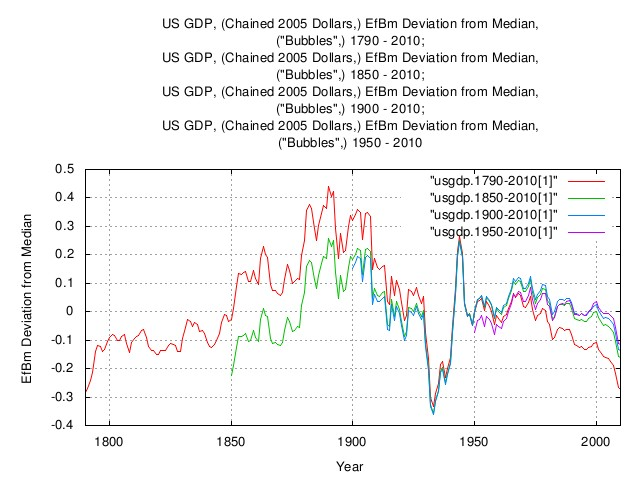Figure III

Figure III is a plot of the EfBm deviation, ("Bubbles,") of the US GDP, chained 2005 dollars, 1790 - 2010, by analyzing the data over different time horizons. The graphs are the median values-note that median, in this case, means half of the time the empirical data is above the LSQ median, and half of the time, below. If the US GDP was a perfect exponential, the graphs all would be a straight line at zero. The graphs are the deviation from the straight line at zero, i.e., the productivity "Bubbles," depending on the time horizon. Note that the graphs, from 1850, 1900, and, 1950 are all quite similar-from 1850 on was the industrialized age; prior to that, the US GDP was, primarily, agricultural and rural. Although the US GDP has geometric Brownian motion characteristics in both eras, the value of the variables changed with industrialization.Figure IV

Figure IV is the same graphs as Figure III, but presents the mean of the EfBm deviation, ("Bubbles,") of the US GDP, chained 2005 dollars, 1790 - 2010, by analyzing the data over different time horizons.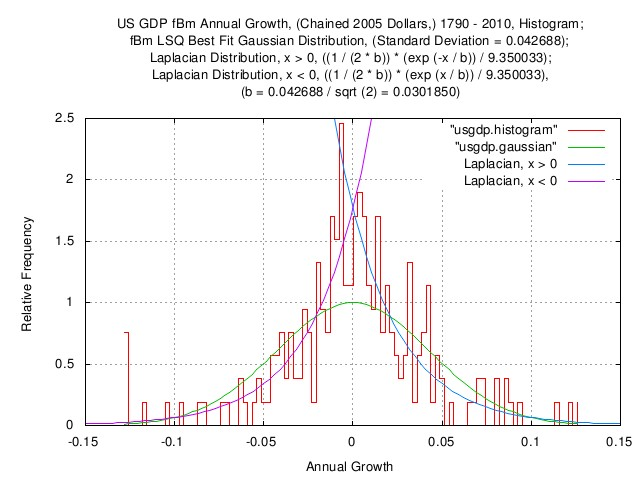Figure V

Figure V is a plot of the fBm, (i.e., marginal increments,) annual growth of the US GDP, chained 2005 dollars, 1790 - 2010, which is consistent with the US GDP having geometric Brownian motion characteristics. Included are LSQ Gaussian/Normal and Laplacian distribution best fits to the histogram. It is important to note the leptokurtosis of the Laplacian distribution-which offers a better approximation to the US GDP dynamics.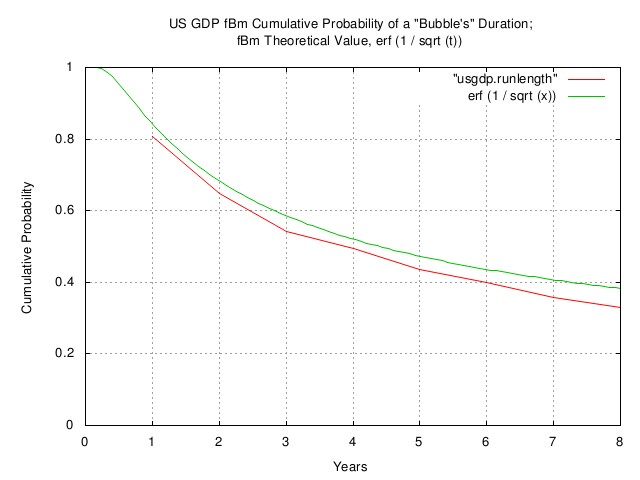Figure VI

Figure VI is a plot of the fBm cumulative probability of the duration of "Bubbles," (i.e., business cycle,) of the US GDP shown in Figure III, and the theoretical value for geometric Brownian motion, ```erf (1 / sqrt (t)```.

 As a side bar, ```erf (1 / sqrt (t)```, for ```t >> 1```, is, approximately, ```1 / sqrt (t)```. Using that approximation, if the US GDP passed through its median value, (zero in Figure III,) what are the chances that the duration of the "Bubble" will be at least 4 years? 50%, (the actual value of `erf (1 / sqrt (4.3)` = 50%.) And, at least 9 years? 1 in 3. A century? 1 in 10, (just like the Gilded Age-about 1850 to 1930-in Figure III.) Note that half of the "Bubbles" will have durations less than 4 years, and half more. It is a difficult concept to grasp, but the formula `erf (1 / sqrt (t)` is true at all time scales, (geometric Brownian motion is a type of fractal that has self similarity at all scales.) The formula is valid for time scales of days, weeks, months, years, centuries ..., (and in algorithmic trading programs, even down to the very small fractions of a second.) The formula has implications for the durations of cultures, also. Why? Because the human birthrate in a culture is greater than unity, which increases the culture's GDP at an exponential rate. Since a culture is subject to random events, (both fortuitous, and not,) it's GDP exhibits dynamics of nonlinear complex system. The dynamics have geometric Brownian motion characteristics. (The geometric properties are created by the nonlinear GDP exponential, the Brownian motion properties are created by the multitude of random events on the GDP.) For some idea of the applicable range of the domain of geometric Brownian motion to culture's GDP, assemble the historical data of how long all cultures lasted in the history of civilization into a histogram; half lasted less than four centuries, half more, (the ancient Egyptians lasted the longest, at 30 centuries.)

Note that the business cycle consists of one positive "Bubble" followed by a negative "Bubble", (or vice versa,) and if the positive, or negative, "Bubble" is "typically" 4.3 years in length, the cycle would be 8.6 years, "typically", which is very close to the 5 years, and, 7 to 11 years often quoted from empirical evidence. (The analysis is reasonably accurate, considering the differences in the eras represented by data spanning nearly quarter of a millennia-about 10% between the theoretical and empirical data.)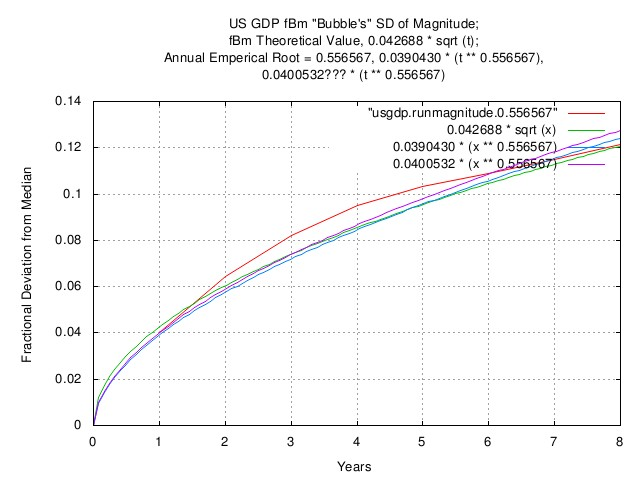Figure VII

Figure VII is a plot of the standard deviation of the magnitude of fBm "Bubbles," (i.e., business cycle,) in the US GDP shown in Figure III, and the theoretical value for geometric Brownian motion, ```rms * sqrt (t)```, where `rms` is one of the two important variables, (the other is `avg`, is the standard deviation of the marginal increments of the GDP, (and `avg` is the average, or mean of the marginal increments.) 67% of the time, the US GDP will be closer to it's median value, (zero in Figure III,) than the value of `rms * sqrt (t)`, and 33% of time, further away from the value of ```rms * sqrt (t)```.

 As a side bar, only two variables, `rms`, the metric of randomness, and, `avg`, the metric of the exponential gain, determine the dynamics of the geometric Brownian motion characteristics-and they are expedient to measure from time series data. The mean, (or time average,) of the marginal returns is `avg`. The square root of the variance, (or standard deviation,) of the marginal returns is `rms`. Then the probability of an "up movement" in any marginal return, `P`, is: `````` avg --- + 1 rms P = ------- ........................................(B-1.24) 2 `````` And, the median gain, `g`, of the marginal returns is: `````` P (1 - P) g = (1 + rms) (1 - rms) ....................(B-1.20) `````` which is maximally optimal when: `````` 2 avg = rms .........................................(B-1.26) `````` which makes: `````` rms = 2 P - 1 ......................................(B-1.18) `````` Interestingly, if ```avg = rms```, and is constant, ```P = 1```, and ```g = (1 + avg)```, which is conceptually similar to simple compound interest. Thus, Geometric Brownian Motion can be conceptualized as compound interest with a random interest rate-which can be negative, creating a "draw down." Note that sum of many Geometric Brownian Motion trajectories, (for example, that make up a GDP,) that the ensemble average (i.e., GDP per capita, having a Log Normal distribution,) is not equal to the time-average of the GDP. Geometric Brownian Motion systems are not ergodic, meaning `g < avg`, (in daily data, by a factor of about 2.)

The randomness in Geometric Brownian Motion systems can be of different forms, too. If the randomness has a Gaussian/Normal distribution, the shape of the graph in Figure VII will be ```rms * sqrt (t) = rms * t^0.5```; but the US GDP is about `rms * t^0.56`, with leptokurtosis, as shown in Figure V. This means the US GDP is slightly more volatile and persistent than the theoretical-but reasonably accurate, considering the differences in the eras represented by data spanning nearly quarter of a millennia, (about 10% at four years between the theoretical and empirical data.)

## Related AnalysisFigure VIII

Figure VIII is a plot of the US GDP, from 1792 to 2010, with LSQ median best fit approximations based on historic data, from 1792 to 2010, 1790 to 1840, and, 1950 to 2000, omitting the effects of the Gilded Age "Bubble," about 1840 through 1950, from the last two. (Note that median, in this case, means half of the time the empirical data is above the LSQ median, and half of the time, below.)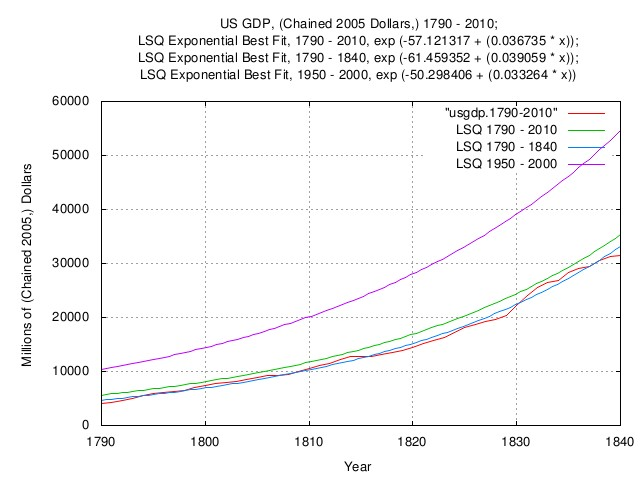Figure IX

Figure IX is an expanded plot of the US GDP in Figure VIII, from 1792 to 1840, with LSQ median best fit approximations based on historic data, from 1792 to 2010, 1790 to 1840, and, 1950 to 2000, omitting the effects of the Gilded Age "Bubble," about 1840 through 1950, from the last two. (Note that median, in this case, means half of the time the empirical data is above the LSQ median, and half of the time, below.)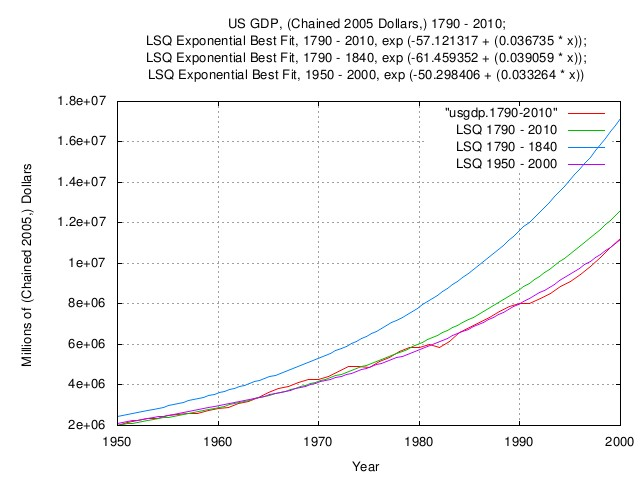Figure X

Figure X is an expanded plot of the US GDP in Figure VIII, from 1950 to 2010, with LSQ median best fit approximations based on historic data, from 1792 to 2010, 1790 to 1840, and, 1950 to 2000, omitting the effects of the Gilded Age "Bubble," about 1840 through 1950, from the last two. (Note that median, in this case, means half of the time the empirical data is above the LSQ median, and half of the time, below.)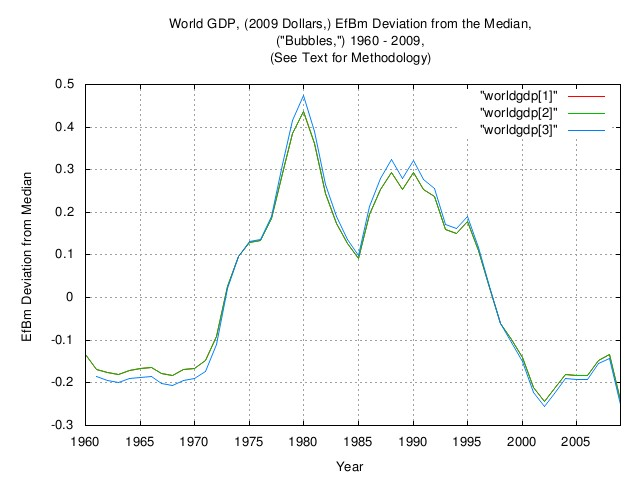Figure XI

Figure XI is a plot of the EfBm deviation, ("Bubbles,") of the World GDP, chained 2009 dollars, 1960 - 2009. If the World GDP was a perfect exponential, the graph would be a straight line at zero. The graph is the deviation from the straight line at zero, i.e., the productivity "Bubbles".

Note that the World GDP has geometric Brownian motion characteristics, and the graphs can be made by three different methods, all of which should produce the same graph, within reason:

•  Exponential LSQ fit of the data.
•  Exponentiation of the linear LSQ of the natural logarithm of the data, and subtracting unity.
•  Exponentiation of the subtraction of the LSQ from the integrated marginal increments of the data, and subtracting unity.

 and , (which are similar,) derive the EfBm deviation "from the outside in," while  "from the inside out"; it is a method of determining that the World GDP has geometric Brownian motion characteristics.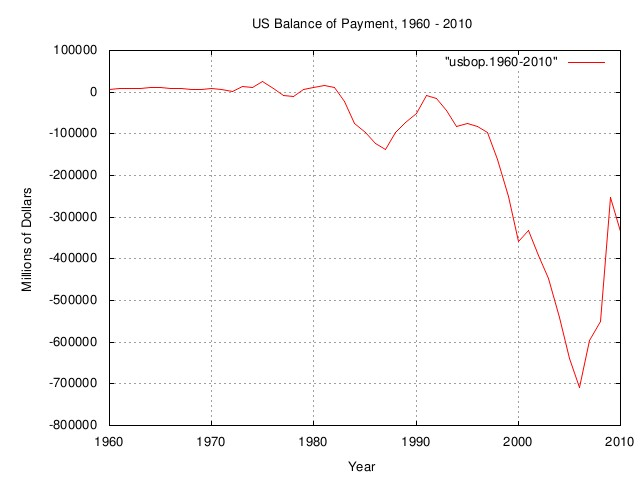Figure XII

Figure XII is a plot of the US balance of payment, 1960 through 2010.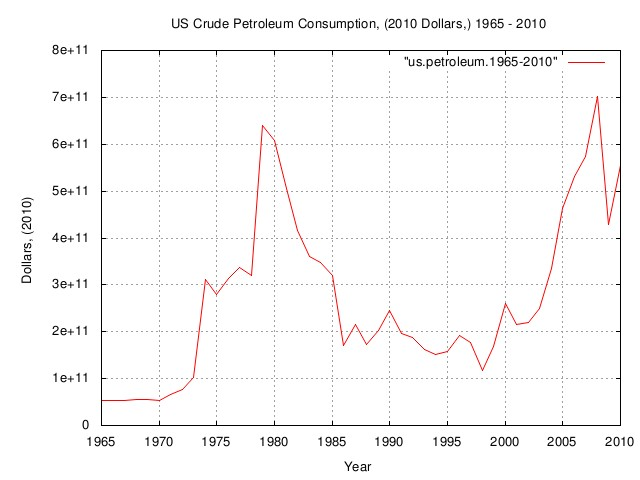Figure XIII

Figure XIII is a plot of the US crude petroleum consumption, 1965 through 2010, in 2010 nominal dollars, and represents that cost of of petroleum energy for the US, an industrialized society.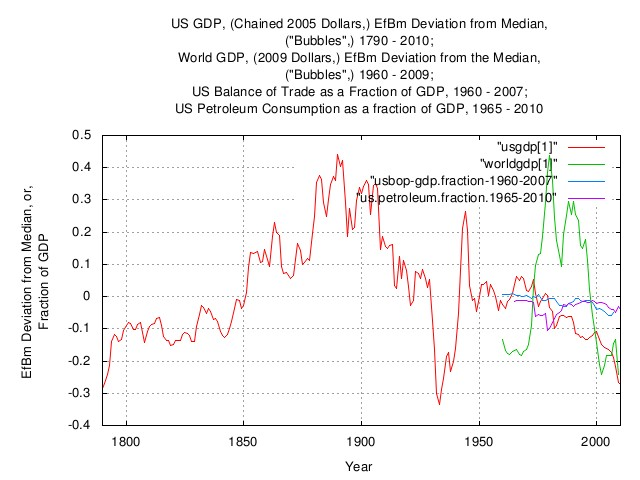Figure XIV

Figure XIV is an overlay plot of the EfBm deviation from the median for various data sets to indicate correlation of "Bubbles".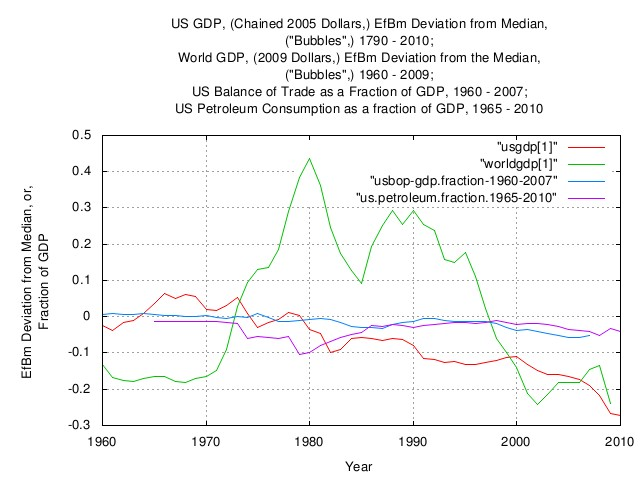Figure XV

Figure XV is expanded overlay plot of the EfBm deviation from the median for various data sets to indicate correlation of "Bubbles", 1960 through 2010.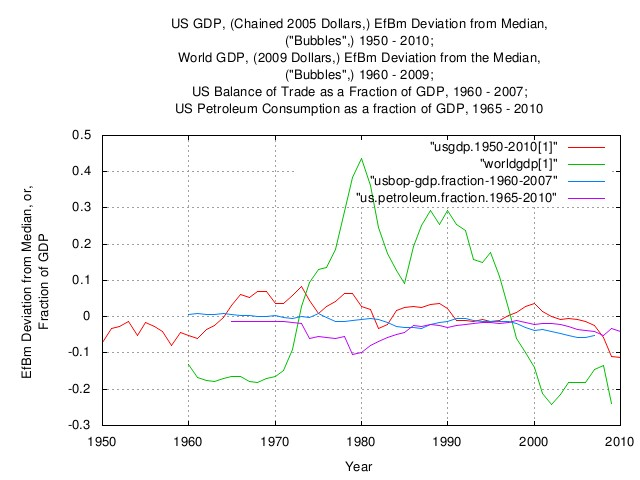Figure XVI

Figure XVI is expanded overlay plot of the EfBm deviation from the median for various data sets to indicate correlation of "Bubbles", 1950 through 2010.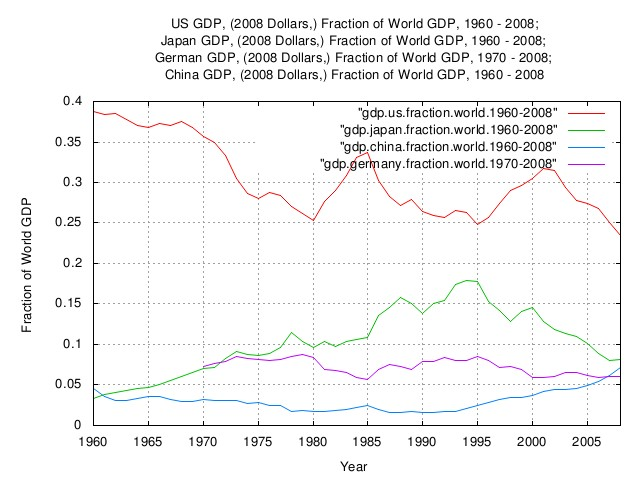Figure XVII

Figure XVII is a plot of the fraction of the World GDP produced by the US, Japan, Germany, and, China, 1960 through 2008. Since 2008, China has become second, surpassing Japan. The graph represents about 2/3 of the World GDP.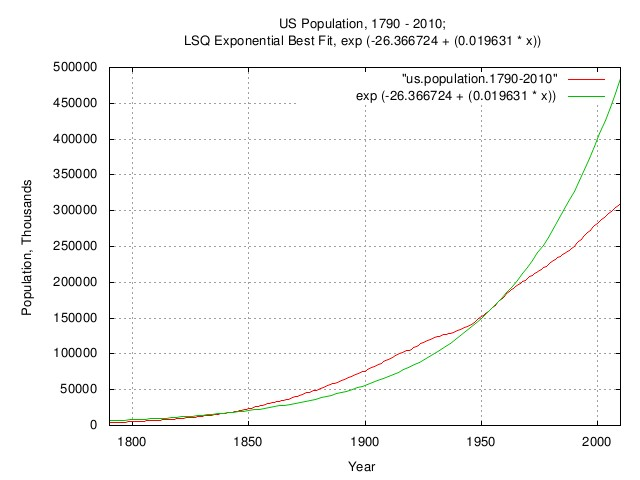Figure XVIII

Figure XVIII is a plot of the US population, 1790 - 2010; and the 1790 - 2010 LSQ median. (Note that median, in this case, means half of the time the empirical data is above the LSQ median, and half of the time, below.)

From 1790 to the present, the average annual increase in US population was about 2.0%, increasing to about 2.3% from 1850 through the early 1900's due to immigration. Starting in the early 1960's, the increase in US population decreased to about 1.1% annually due to family planning. Interestingly, during the Great Depression, 1929 through 1939, the birth rate decreased to about 1%, increasing to about 2.3% in the era of the post WWII "Baby Boomers."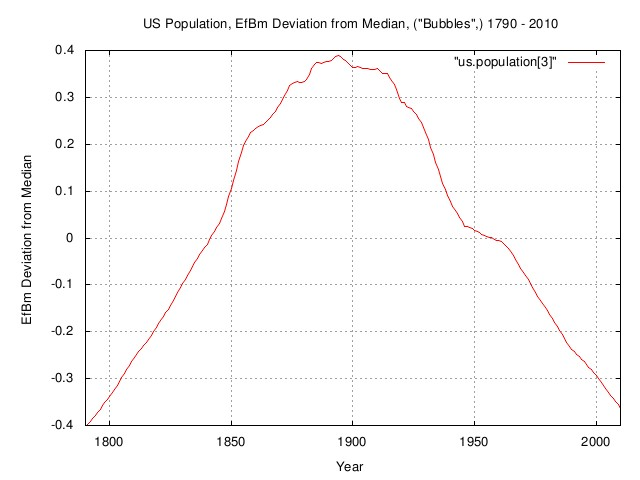Figure XIX

Figure XIX is a plot of the EfBm deviation from the LSQ median, "Bubbles," in the US population, 1790 through 2010. (Note that median, in this case, means half of the time the empirical data is above the LSQ median, and half of the time, below.) Immigration in the last half of the 1800's increases the value of the median, decreasing both ends of the LSQ. Starting in the early 1960's, the increase in US population decreased to about 1.1% annually due to family planning. Interestingly, during the Great Depression, 1929 through 1939, the birth rate decreased to about 1%, increasing to about 2.3% in the era of the post WWII "Baby Boomers."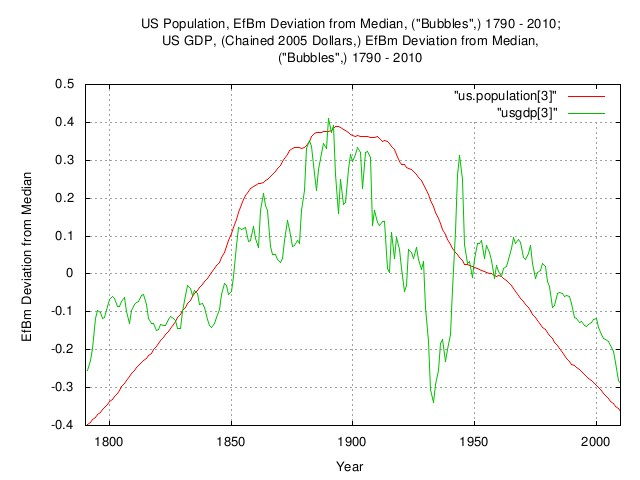Figure XX

Figure XX is an overlay plot of Figure II, the EfBm deviation, ("Bubbles,") of the US GDP, chained 2005 dollars, 1790 - 2010, and, the previous graph, Figure XIX, the EfBm deviation from the LSQ median, "Bubbles," in the US population, 1790 through 2010.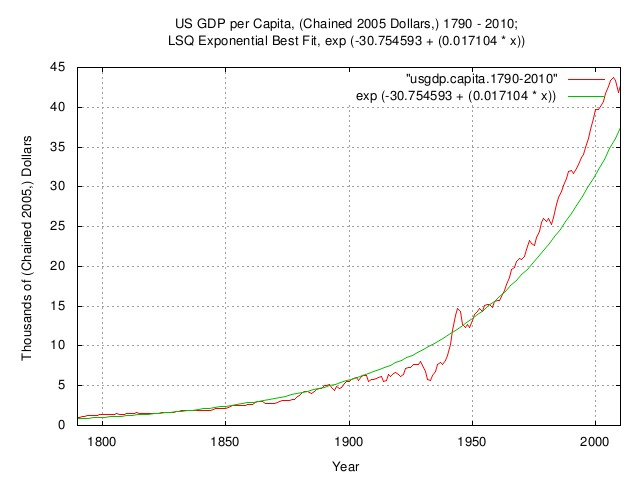Figure XXI

Figure XXI is a plot of the US population, 1790 - 2010; and the 1790 - 2010 LSQ median. (Note that median, in this case, means half of the time the empirical data is above the LSQ median, and half of the time, below.)

From 1790 to the present, the average annual increase in US population was about 2.0%, increasing to about 2.3% from 1850 through the early 1900's due to immigration. Starting in the early 1960's, the increase in US population decreased to about 1.1% annually due to family planning. Interestingly, during the Great Depression, 1929 through 1939, the birth rate decreased to about 1%, increasing to about 2.3% in the era of the post WWII "Baby Boomers."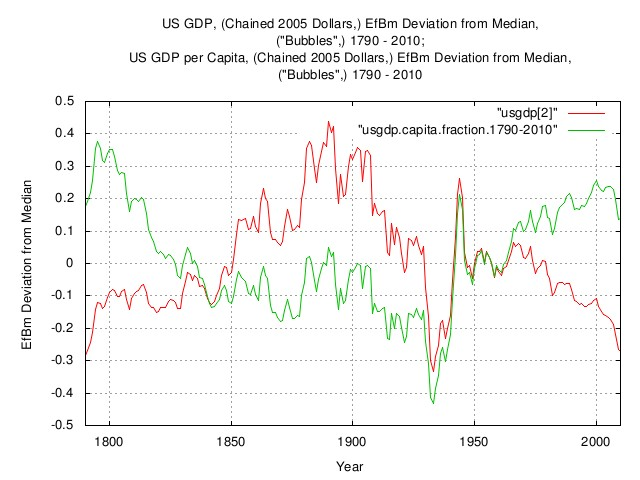Figure XXII

Figure XXII is an overlay plot of the previous graph's, (Figure XXI,) EfBm deviation and the EfBm deviation, ("bubbles,") of the US GDP per capita, chained 2005 dollars, 1790 - 2010. If the US GDP per capita was a perfect exponential, the graph would be a straight line at zero. The graph is the deviation from the straight line at zero, i.e., the productivity "Bubbles".

## Archive

The data presented here can be reconstructed, in its entirety, from the historical.economics.tar.gz tape archive file, which contains all data and references, thereto. The source code to all programs used in the analysis is available from the NtropiX site, tsinvest.tar.gz, and, the NdustrixX site, fractal.tar.gz, tape archive files. All use the "standard" Unix development systems of rcs(1) and make(1) to facilitate replication.

It should be noted that many of the data set sizes are pitifully small-some as few as two hundred data points, (a standard error of about 7% of the standard deviation,) and conclusions can only be regarded as circumstantial.

The information contained herein is private and confidential and dissemination is strictly forbidden, except under the provisions of contractual license.

THE AUTHOR PROVIDES NO WARRANTIES WHATSOEVER, EXPRESSED OR IMPLIED, INCLUDING WARRANTIES OF MERCHANTABILITY, TITLE, OR FITNESS FOR ANY PARTICULAR PURPOSE. THE AUTHOR DOES NOT WARRANT THAT USE OF THIS INFORMATION DOES NOT INFRINGE THE INTELLECTUAL PROPERTY RIGHTS OF ANY THIRD PARTY IN ANY COUNTRY.

So there.

Comments, questions, and problem reports should be addressed to:

john@email.johncon.com

http://www.johncon.com/john/
http://www.johncon.com/ntropix/
http://www.johncon.com/ndustrix/
http://www.johncon.com/nformatix/
http://www.johncon.com/ndex/